﻿ A Glass Vessel Insulating Cup GVIC Calorimeter to Study Chemical Reactions in the Liquid PhaseJournal of Laboratory Chemical Education

p-ISSN: 2331-7450    e-ISSN: 2331-7469

2022;  10(1): 4-8

doi:10.5923/j.jlce.20221001.02

Received: Mar. 10, 2022; Accepted: Mar. 25, 2022; Published: Apr. 15, 2022### A Glass Vessel Insulating Cup GVIC Calorimeter to Study Chemical Reactions in the Liquid Phase

Tarek R. Farhat, Hasan El Rifai, Rana Jisr

Physical Sciences, West Virginia University Institute of Technology, Beckley, WV, USA

Correspondence to: Tarek R. Farhat, Physical Sciences, West Virginia University Institute of Technology, Beckley, WV, USA.
 Email: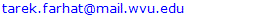Abstract

An alternative setup to the classical cup calorimetry to monitor simple chemical reactions in the liquid phase is introduced and discussed. One of the most common calorimetry procedures used in college chemistry labs is the foam or coffee cup reactor. In this paper, we will test and evaluate a Glass Vessel Insulating Cup (GVIC) Calorimeter composed of parts readily available in labs but inherently different in design from a regular cup calorimeter. Results obtained were well within the statistical limit and the GVIC technique saved more than 5 times on solution consumption. The reaction system studied is the enthalpy of neutralization using a dilute alkaline solution of NaOH and acidic solutions of either HCl or H2SO4 solutions.

Keywords: Aqueous Solution Chemistry, Calorimetry, Thermochemistry, Neutralization, Enthalpy

Cite this paper: Tarek R. Farhat, Hasan El Rifai, Rana Jisr, A Glass Vessel Insulating Cup GVIC Calorimeter to Study Chemical Reactions in the Liquid Phase, Journal of Laboratory Chemical Education, Vol. 10 No. 1, 2022, pp. 4-8. doi: 10.5923/j.jlce.20221001.02.

### 1. Introduction

Extensive Thermodynamic properties like enthalpy change ∆H and entropy change ∆S of a reaction are studied in academic labs using simple apparatus. Other thermodynamic quantities such as Gibbs free energy change ∆G and equilibrium constant Kc of a reaction are obtained from ∆H and entropy change ∆S [1,2,3]. Most popular calorimetry/thermochemistry experiments are dissolution, neutralization, and dilution reactions [1,2,3]. In most experiments heat is either liberated or absorbed and the rise or drop in temperature is monitored using a calorimeter setup. Any Temperature increase is indicative of an exothermic process which in general associates with –∆H or –∆G. Students usually perform experiments to measure the heat capacity of the cup calorimeter, Cc, otherwise, it will be provided as an approximate measure [1,2]. A simple and cheap cup-calorimeter setup conducted at high schools and undergrad/general chemistry levels would use foam cups, polystyrene cups, or paper coffee cups as the core of the experiment [1,2,4,5,6]. The objective of the published setups is to seek low cost and easy to assemble chemistry experiments for chemical education [7,8,9,10]. In most cases cups come without a cover, thus, cardboard lids with a hole are used instead. A hole in the lid is made to accommodate the thermometer. Care must be taken not to puncture the foam or paper cup when stirring or fitting the thermometer. To avoid using the approximate value of the heat capacity, Cc, of the cup-calorimeter, students can spend half the lab’s time working to get the temperature profile of the hot-cold water mixture , though the use of electronic equipment reduced it to a few minutes . To achieve a certain level of acceptable accuracy, a cup-Calorimeter would need at least 50 mL of each liquid to run the experiment. That is, the cup reactor can easily accommodate a 100 mL mixture. A typical class setting of 20 students would need at least 1.0 liter of acid solution and 1 liter of the base solution. Normally, two acids (e.g. HCl, H2SO4) are used, making the volume required up to 2 liters each. A prudent Lab supervisor would prepare double the quantity to account for solution losses and repetition of experimental procedures which requires 2L of acid solution HCl, 2L of acid solution H2SO4, and 4L of NaOH solution. A problem arises at universities, colleges, and high schools of multiple sections, where volumes can easily exceed 15 liters for general chemistry lab prep work to conduct experiments such as neutralization reactions. Handling such large volumes is inconvenient to lab managers and supervisors.
In this paper, we propose using easy to assemble Calorimeter that we call a “Glass Vessel Insulating Cup” (GVIC) Calorimeter where temperature can be accurately monitored while solution volume consumption is cut off by four to five times. Note, although a foam cup is used it is not the reactor. The purpose of the foam cup or any cup is to add another layer of insulation to the glass vessel which is the reactor. Liquids are poured into the glass vessel and not the foam cup. With a glass vessel, most solutions or liquids commonly used in educational laboratories can be poured inside the glassy reactor to study different types of chemical reactions in the liquid phase. For example, the GVIC can study reactions of liquids that would otherwise dissolve the foam cup. Thus, widens the versatility of the proposed method to organic liquids rather than aqueous liquids only. The GVIC heat capacity is accurately determined by weighing the glass vessel so no time is wasted on finding, Cc, of a calorimeter. Our CHEM115L students used the GVIC to determine the enthalpy of neutralization reaction: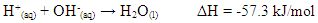In most experiments conducted by our students, temperature changes of the reaction mixture exceeded 5°C and were accurately measured to determine enthalpy changes.

### 2. Experimental Procedure

Under this section, we will discuss hardware and chemicals, logistics, the heat capacity of the calorimeter, calorimeter setup, and hazards.

#### 2.1. Chemicals and Hardware

Chemicals were used from the supplier without any further processing. Reagent NaOH 99% pearls from Alfa-Aesar, HCl acid 37%, and sulfuric acid 98% from Sigma. Solutions of 2.0 M HCl, 2.0M NaOH, and 1.0 M H2SO4 were prepared using DI water, analytical balance, grad cylinders, and volumetric flasks. Acid solutions were stored in glass bottles while NaOH solutions were in plastic bottles ready for students’ use. Foam cups, cotton plugs, glass bottles (1 oz), digital thermometers, and aluminum foil are part of the normal equipment in our laboratories and were used as-is.

#### 2.2. Logistics and Procedure

The “Glass Vessel Insulating Cup” (GVIC) Calorimeter experiment was performed by two different groups. The first group comprised teaching assistants at West Virginia University Institute of Technology (WVU-Tech) who obtained and verified results independent from students taking the CHEM115L course. The 2nd group comprised undergrad students of the CHEM115L course at WVU-Tech registered in various sections and semesters.

#### 2.3. Heat Capacity of the Calorimeter

In the first part of the experiment, the glass vessel is either a 30 mL glass bottle (1oz bottle) or 25 mL Erlenmeyer flask, Figure-1 (a-i, a-ii). The glass bottle (~ 3 mm wall thickness) was weighed either using a top-loading balance of a tolerance ±0.01 g or an analytical balance with 100 g capacity. The mass of the glass bottle, Mgl, can be used later to determine the heat capacity of the calorimeter, Ccal. Next, is to prepare the calorimeter setup.

#### 2.4. Calorimeter Setup to Monitor Temperature Change

(i) Parts needed: Cotton plugs, foam cup, and aluminum foil for insulation and support. Two glass bottles with a one-hole rubber stopper. A digital thermometer to monitor temperature change of the neutralization reaction.
(ii) Procedure: Cotton plugs are placed at the bottom of the foam cup to act as a cushion. The glass bottle or flask must be covered with aluminum foil wrapped around it in two layers, Figure-1 (a-iii).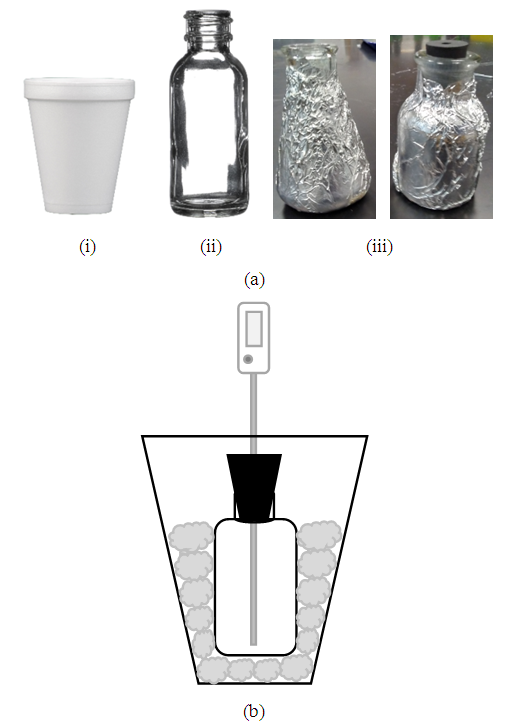Figure 1. Apparatus used to conduct the “Glass Vessel Insulating Cup” experiment; (i) foam cup, (ii) glass bottle, (iii) glass bottle insulated with aluminum foil, (b) Schematic of the GISC setup where the glass bottle is enclosed inside the foam cup surrounded by cotton plugs. The digital thermometer is held by the rubber stopper
The glass bottle resting on the cotton cushion was surrounded with more cotton plugs to cover the bottle to the rim, Figure-1b. The GVIC Calorimeter is now ready to take the acid solution.
In this experiment, students would use another foam cup with Aluminum-insulated (1 oz glass bottle or a 25 mL Erlenmeyer flask) to accommodate the second solution which is the NaOH solution. For a group of two students, one student can Dispense 10.0 mL of the assigned acid (i.e. HCl or H2SO4) solution at room temperature from a 10.0 mL pipette into the (GVIC) Calorimeter. The other student can dispense 10.0 mL of the base NaOH solution, at room temperature, into the other insulated flask or bottle using a 10.0 mL pipette or a plastic 10.0 mL syringe. Students should swirl gently the mixture inside the calorimeter glass bottle (Note: for concentrated acid or base solutions always pour the concentrated over the dilute solution). Rubber stoppers can be loosened slightly to relieve pressure if deemed necessary. Digital thermometers can be used to monitor the temperature of the acid solution and the NaOH solution at an accuracy of ±0.01°C. Unlike a foam cup, whether a 1 oz glass bottle or a 25 mL Erlenmeyer flask, these can be sealed with rubber stoppers that hold respective thermometers. Students would measure and record the temperature directly on a spreadsheet, Temperature (°C) vs time(s) data for each of the two solutions (i.e. acid solution and NaOH solution) are recorded at 30-s intervals for ~ 12 to 15-mins period. If two students are working as partners, one student can read the temperature while the other student records the temperature measurement. The plateau of the curve obtained after plotting the results represents the final temperature Tfinal (check later). On the electronic report, a plot is automatically generated allowing students to follow the progress of their measurements. Lab managers and instructors might find it more suitable to use big plastic or metallic cups as an insulating container for the small glass vessel to conduct this experiment.

#### 2.5. Hazards

Hydrochloric acid HCl, sulfuric acid H2SO4, and sodium hydroxide NaOH reagent bottles contain corrosive chemicals and should be handled with care. A prudent measure is to prepare respective dilute solutions of all reagents using the fume hood, gloves, aprons, and goggles. Always pour concentrated liquids of acids over pure water in volumetric flasks. After reaching the dilution mark in a volumetric flask, freshly prepared acid solutions tend to shrink in volume and overheat. Keep checking on the mark and add more pure water when the level drops below it. With gloves on, homogenize the mixture after each addition of pure water. All solutions of NaOH should be stored in PP or PE bottles. Dilute solutions of 2.0 M NaOH, 2.0 M HCl, and 1.0 M H2SO4 can be used on Lab benches to conduct enthalpy change experiments using full gear of PPE.

### 3. Results and Discussion

In Part I of this experiment, students would determine the calorimeter constant of the “Glass Vessel Insulating Cup (GVIC)” calorimeter”. To a first approximation, the surrounding of the neutralization reaction in aqueous media is confined to the glass container only. The foam cup, rubber stopper, and cotton insulation are not included because these are not in direct contact with the liquid and their contribution to heat capacity is negligible. The mass of insulating aluminum foil is in the range of 1.5 to 2.5 g with a specific heat capacity, sAl, of 0.9 Jg-1°C-1. The specific heat capacity of soft glass, sgl, is 0.84 Jg-1°C-1 and the mass range of the glass containers (i.e. glass bottles or jars, flasks,.. etc.) is between 55.0 g to 65.0 g. If the mass of aluminum foil is ignored it will introduce an error of 3.5%±0.5%. Instructors are welcome to add the contribution of the insulation material mentioned per their lab setup. After the mass of the glass bottle, Mgl, is measured students were able to calculate the approximate heat capacity of Calorimeter, Ccal, such that,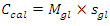(1)
In Part II, the heat of the reaction, Q, is determined by measuring the sudden change in temperature, ∆T = Tfinal – Tinitial. The term Tfinal is the temperature of the hot salt solution after mixing while Tinitial is the temperature of the acid solution or base solution in the reactor before mixing, Figure-2. Normally, the ambient temperature of either the acid or base solutions in separate bottles should be the same otherwise the temperature of the solution or liquid in the glass vessel reactor should be recorded. In similar academic Labs, students make an approximation by assuming the specific heat of the solution is the same as the specific heat capacity of water and use sw = 4.18 J/g°C. However, in our experiments we used the specific heat of the salt solutions, ssol, (e.g. s(Na2SO4) = 3.8 Jg-1°C-1 and the solution density dsol = 1.09 g/mL, s(NaCl) = 3.89 Jg-1°C-1 and the solution density dsol = 1.04 g/mL) . Thus, the total heat of the reaction, Qt, is obtained by adding heat gained by the solution mixture to the heat gained by GVIC such that,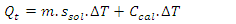(2)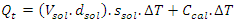(3)
where Vsol volume of salt solution. Therefore, enthalpy of neutralization is determined from,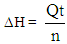(4)
n is the amount in moles of the limiting reactant.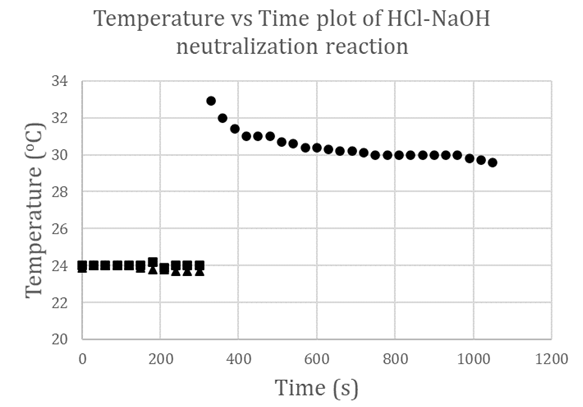Figure 2. A plot of Temperature (°C) vs time (s) of the reaction of 2.0 M HCl solution with 2.0 M NaOH solution starting at an ambient temperature of 24°C. Around t = 300 s, the acid/base solutions were mixed causing a sharp temperature rise to 33°C that stabilized to 30°C for a time interval of 150 s
From thermal plots like in Figure-2 students are instructed to quickly record the temperature sudden increase after mixing. The gradual decrease in temperature until it stabilizes to a plateau is monitored. Temperature change ΔT was determined by extrapolating a straight line that traverses the maximum number of points through the plateau region and intersects the temperature axis (e.g. ∆T ~ 6°C from the HCl-NaOH plot). Another illustration is given in Figure-3 where ∆T ~ 8°C from an H2SO4-NaOH plot was determined. Similar plots can be obtained of any type of exothermic or endothermic reaction irrespective of the liquid chemicals under study provided these chemicals do not corrode soft glass. For example, instructors of a physical organic chemistry lab can study the enthalpy of oxidation of a ketone with chromic acid. These chemicals are detrimental to foam and paper cups, but this oxidative-thermodynamic reaction can be safely studied using the GVIC calorimeter.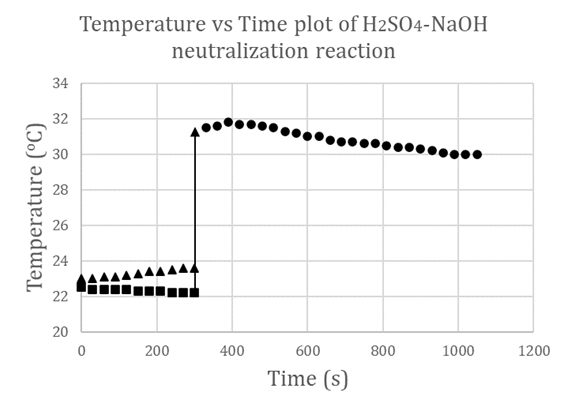Figure 3. A plot of Temperature (°C) vs time (s) of the reaction of 1.0 M H2SO4 solution with 2.0 M NaOH solution starting at an ambient temperature of ~ 23°C. Around t = 300 s, the acid/base solutions were mixed causing a sharp temperature rise to ~ 32°C that stabilized to 31°C for a time interval of 120 s

#### 3.1. Method Validation

Using equations (1) to (4), we can calculate values of heat gained by solution mixture, Qmix, and the heat gained by calorimeter, QCal, thus be able to obtain the total heat of the reaction, Qt, in J. The enthalpy of neutralization ΔH can be determined experimentally per the amount of acid or base used. To validate the proposed experimental method, we used two sources of data. The first source, experiments were carefully conducted and monitored for reproducibility by teaching assistants where their results were used as a reference, while the second source is the data gathered from CHEM115L students’ electronic reports. We managed to gather a population of twenty-two independent data sets. For the enthalpy of neutralization experiment, the two parameters that were considered for statistical analysis are the temperature rise ΔT (°C) and the enthalpy change ΔH (kJ/mol). The mean ū and the standard deviation σ of ΔTs and ΔHs of these reports were calculated. From the first source, experiments were carefully conducted to minimize errors, so these were considered our standard reference frame. The reference temperature change determined was ΔTr = 7.4°C and the corresponding enthalpy change is ΔHr = 51.67 kJ/mol. From the second source, where data is expected to scatter and fluctuate according to students’ performance, the mean ū and the standard deviation σ are calculated using a spreadsheet and conveniently presented in Table 1.
 Table 1. Statistical parameters of ū and σ related to physical parameters ΔTr and ΔHr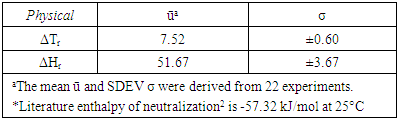#### 3.2. The t-test

For a normal distribution of ΔTs values, a reasonable range of temperature change was obtained between 4 to 11°C. In terms of %population, ~68% of the data points fall within ū ± 1.σ and ~95% fall within ū ± 2.σ range. The confidence interval CI of a t-test where t = 2.08 at 95% confidence level and n -1 = 21 degrees of freedom is determined from,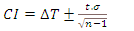(5)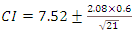(6)
The CI range of 7.25 to 7.79 contains ΔTr = 7.40°C reference value which correlates with the work conducted by our TAs under controlled conditions.
For a normal distribution of ΔHs values, a reasonable range of enthalpy change was obtained between 35 to 65 kJ/mol. In terms of %population, ~68% of the data points fall within ū ± 1.σ and ~95% fall within ū ± 2.σ range. The confidence interval CI of a t-test where t = 2.08 at 95% confidence level and n -1 = 21 degrees of freedom is calculated from equation-5 and Table 1,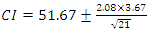(7)
The CI range of 50.00 to 53.33 contains ΔHr = 51.67 kJ/mol reference which correlates with the work conducted by our TAs under controlled conditions. The % difference between students’ average of ΔH using the GVIC technique and the literature ΔH is ~9.8%. Note, 6% of our CHEM115L students’ reports obtained ΔH values that were ≤ 5% different from the literature value of -57.32 kJ/mol. The difference in enthalpy values between students’ experiments and the literature can be attributed to many reasons. A major factor is students’ random technical skills using labware, followed by heat losses, not including the heat capacity of insulating layers, and the GVIC technique is not expected to be as accurate as a commercial calorimeter. This statistical analysis that relied on gathering data from WVU-Tech student e-lab reports has demonstrated that the GVIC calorimetric method compared to the conventional [foam, polystyrene, paper, …etc.] coffee cup laboratory methods are viable and more adaptable. The GVIC method with a capability to handle a variety of chemicals and solutions using 4 to 5 times smaller volumes used by comparable cup-methods would open the door for educators in the chemistry domain to propose new labs in thermodynamics and kinetics principles.

### 4. Conclusions

In this paper, we have introduced a technical modification to the conventional foam or coffee cup calorimeter, that is the “Glass Vessel Insulating Cup” (GVIC) calorimeter to study simple laboratory chemical reactions. The proposed experimental technique uses insulated small glass vessels thus offering many advantages. The GVIC (i) shortens the time of the experiment, (ii) makes ~5 times saving on solutions expenditure, (iii)hence less waste to dispose of, (iv) allows a greater variety of chemicals and solutions to react in a glass reactor where polystyrene, foam, or paper cup fails, (v) uses apparatus that is simple to assemble in any educational lab.

### ACKNOWLEDGEMENTS

The authors would like to thank all students of West Virginia University Institute of Technology who contributed to this work directly or indirectly.

### References

  Lawrence N. Ngeh, John D. Orbell, and Stephen W. Bigger, 1994, Simple heat flow measurements: a closer look at Polystyrene cup calorimeters J. Chem. Educ., 71, 9, 793.  Fundamentals of Chemistry Lab Manual, Enthalpy Changes in Chemical Reactions: Hess’s law, West Virginia Institute of Technology, Beckley, WV 2019/2020.  Frazier Nyasulu, Rebecca Barlag, Lindy Wise, and Lauren McMills, 2013, The H3PO4 acid ionization reactions: a capstone multi-concept thermodynamics general chemistry laboratory exercise J. Chem. Educ., 90, 5, 642–645.  A. M. R. P. Bopegedera and K. Nishanthi R. Perera†, 2017 “Greening” a familiar general chemistry experiment: coffee cup calorimetry to determine the enthalpy of neutralization of an acid–base reaction and the specific heat capacity of metals J. Chem. Educ., 94, 4, 494–499.  Albert W. Burgstahler and Clark E. Bricker, 1991, Measuring the heat of sublimation of dry ice with a polystyrene foam cup calorimeter J. Chem. Educ., 68, 4, 332.  John J. Stankus and Jennifer D. Caraway, 2011, Replacement of coffee cup calorimeters with fabricated beaker calorimeters J. Chem. Educ., 88, 12, 1730-1731.  Emma Kavanagh, Sam Mindel, Giles Robertson, and D. E. Peter Hughes, 2008, An inexpensive solution calorimeter, J. Chem. Educ., 85, 8, 1129.  Luis Alfonso Torres-Gomez and Aaron Rojas-Aguilar, 1991, A low-cost labyrinth calorimeter for reaction-solution experiments J. Chem. Educ., 68, 8, 671.  Ben Ruekberg, An economical, safe, and sturdy student calorimeter, 1994, J. Chem. Educ., 71, 4, 333.  Henry Brouwer, Small-scale thermochemistry experiment, 1991, J. Chem. Educ., 68, 7, A178.  Rebecca E. Barlag, Phyllis Arthasery, and Frazier Nyasulu, 2010, Electrical Determination of the Heat Capacity of a Calorimeter in Approximately One Minute, J. Chem. Educ., 87, 9, 992.